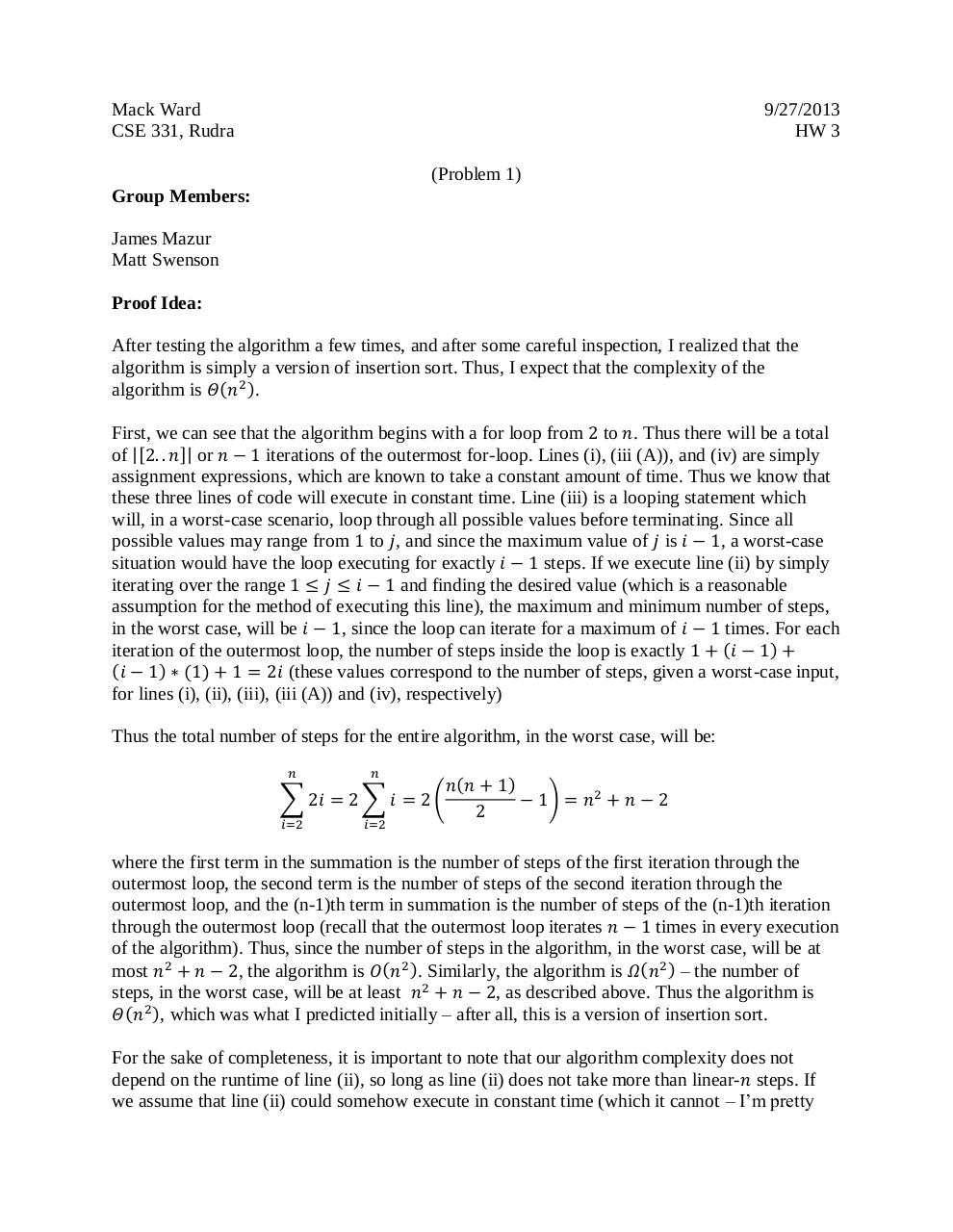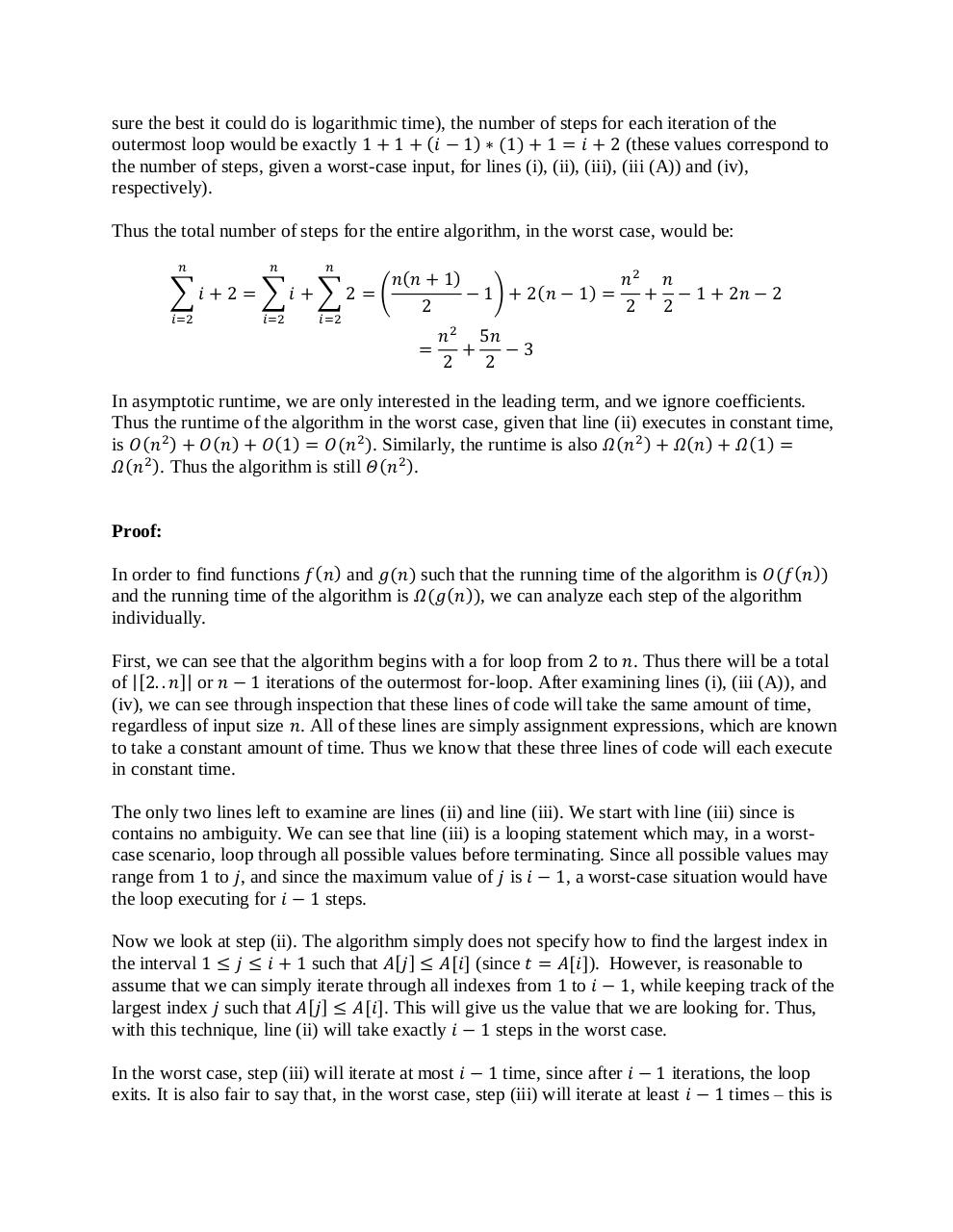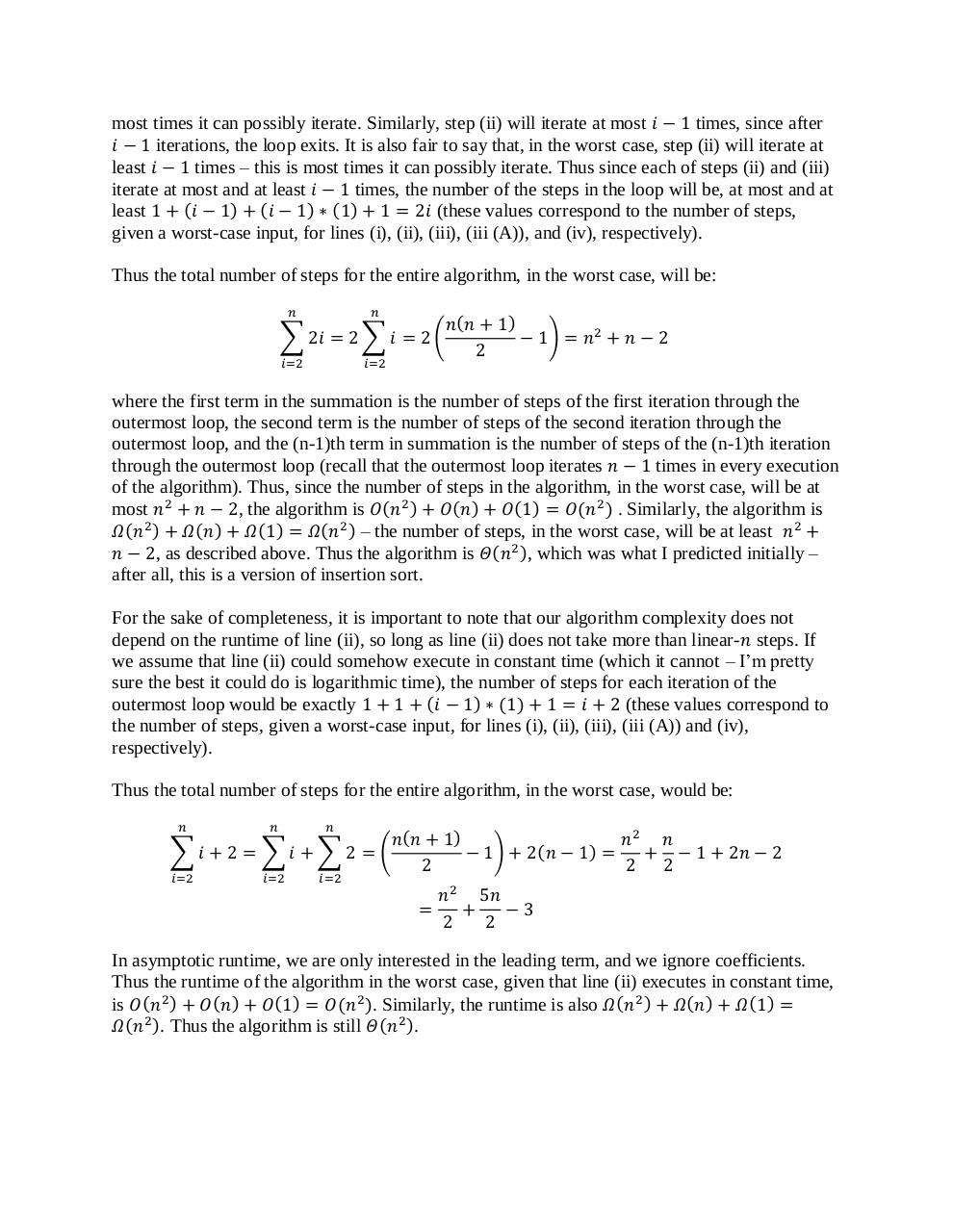# HW 3.1 .pdf

### File information

Original filename: HW 3.1.pdf
Author: Mack Ward

This PDF 1.5 document has been generated by MicrosoftÂ® Word 2013, and has been sent on pdf-archive.com on 15/10/2013 at 19:47, from IP address 67.20.x.x. The current document download page has been viewed 962 times.
File size: 194 KB (3 pages).
Privacy: public file

HW 3.1.pdf (PDF, 194 KB)

### Document preview

Mack Ward
CSE 331, Rudra

9/27/2013
HW 3
(Problem 1)

Group Members:
James Mazur
Matt Swenson
Proof Idea:
After testing the algorithm a few times, and after some careful inspection, I realized that the
algorithm is simply a version of insertion sort. Thus, I expect that the complexity of the
algorithm is 𝛩(𝑛2 ).
First, we can see that the algorithm begins with a for loop from 2 to 𝑛. Thus there will be a total
of |[2. . 𝑛]| or 𝑛 − 1 iterations of the outermost for-loop. Lines (i), (iii (A)), and (iv) are simply
assignment expressions, which are known to take a constant amount of time. Thus we know that
these three lines of code will execute in constant time. Line (iii) is a looping statement which
will, in a worst-case scenario, loop through all possible values before terminating. Since all
possible values may range from 1 to 𝑗, and since the maximum value of 𝑗 is 𝑖 − 1, a worst-case
situation would have the loop executing for exactly 𝑖 − 1 steps. If we execute line (ii) by simply
iterating over the range 1 ≤ 𝑗 ≤ 𝑖 − 1 and finding the desired value (which is a reasonable
assumption for the method of executing this line), the maximum and minimum number of steps,
in the worst case, will be 𝑖 − 1, since the loop can iterate for a maximum of 𝑖 − 1 times. For each
iteration of the outermost loop, the number of steps inside the loop is exactly 1 + (𝑖 − 1) +
(𝑖 − 1) ∗ (1) + 1 = 2𝑖 (these values correspond to the number of steps, given a worst-case input,
for lines (i), (ii), (iii), (iii (A)) and (iv), respectively)
Thus the total number of steps for the entire algorithm, in the worst case, will be:
𝑛

𝑛

∑ 2𝑖 = 2 ∑ 𝑖 = 2 (
𝑖=2

𝑖=2

𝑛 (𝑛 + 1)
− 1) = 𝑛2 + 𝑛 − 2
2

where the first term in the summation is the number of steps of the first iteration through the
outermost loop, the second term is the number of steps of the second iteration through the
outermost loop, and the (n-1)th term in summation is the number of steps of the (n-1)th iteration
through the outermost loop (recall that the outermost loop iterates 𝑛 − 1 times in every execution
of the algorithm). Thus, since the number of steps in the algorithm, in the worst case, will be at
most 𝑛2 + 𝑛 − 2, the algorithm is 𝑂(𝑛2 ). Similarly, the algorithm is 𝛺(𝑛2 ) – the number of
steps, in the worst case, will be at least 𝑛2 + 𝑛 − 2, as described above. Thus the algorithm is
𝛩(𝑛2 ), which was what I predicted initially – after all, this is a version of insertion sort.
For the sake of completeness, it is important to note that our algorithm complexity does not
depend on the runtime of line (ii), so long as line (ii) does not take more than linear-𝑛 steps. If
we assume that line (ii) could somehow execute in constant time (which it cannot – I’m pretty

sure the best it could do is logarithmic time), the number of steps for each iteration of the
outermost loop would be exactly 1 + 1 + (𝑖 − 1) ∗ (1) + 1 = 𝑖 + 2 (these values correspond to
the number of steps, given a worst-case input, for lines (i), (ii), (iii), (iii (A)) and (iv),
respectively).
Thus the total number of steps for the entire algorithm, in the worst case, would be:
𝑛

𝑛

𝑛

𝑖=2

𝑖=2

𝑖=2

𝑛(𝑛 + 1)
𝑛2 𝑛
(
)
∑𝑖 + 2 = ∑𝑖 + ∑2 = (
− 1) + 2 𝑛 − 1 =
+ − 1 + 2𝑛 − 2
2
2 2
𝑛2 5𝑛
=
+
−3
2
2

In asymptotic runtime, we are only interested in the leading term, and we ignore coefficients.
Thus the runtime of the algorithm in the worst case, given that line (ii) executes in constant time,
is 𝑂(𝑛2 ) + 𝑂(𝑛) + 𝑂(1) = 𝑂(𝑛2 ). Similarly, the runtime is also 𝛺 (𝑛2 ) + 𝛺(𝑛) + 𝛺(1) =
𝛺(𝑛2 ). Thus the algorithm is still 𝛩(𝑛2 ).

Proof:
In order to find functions 𝑓 (𝑛) and 𝑔(𝑛) such that the running time of the algorithm is 𝑂(𝑓(𝑛))
and the running time of the algorithm is 𝛺(𝑔(𝑛)), we can analyze each step of the algorithm
individually.
First, we can see that the algorithm begins with a for loop from 2 to 𝑛. Thus there will be a total
of |[2. . 𝑛]| or 𝑛 − 1 iterations of the outermost for-loop. After examining lines (i), (iii (A)), and
(iv), we can see through inspection that these lines of code will take the same amount of time,
regardless of input size 𝑛. All of these lines are simply assignment expressions, which are known
to take a constant amount of time. Thus we know that these three lines of code will each execute
in constant time.
The only two lines left to examine are lines (ii) and line (iii). We start with line (iii) since is
contains no ambiguity. We can see that line (iii) is a looping statement which may, in a worstcase scenario, loop through all possible values before terminating. Since all possible values may
range from 1 to 𝑗, and since the maximum value of 𝑗 is 𝑖 − 1, a worst-case situation would have
the loop executing for 𝑖 − 1 steps.
Now we look at step (ii). The algorithm simply does not specify how to find the largest index in
the interval 1 ≤ 𝑗 ≤ 𝑖 + 1 such that 𝐴[𝑗] ≤ 𝐴[𝑖] (since 𝑡 = 𝐴[𝑖]). However, is reasonable to
assume that we can simply iterate through all indexes from 1 to 𝑖 − 1, while keeping track of the
largest index 𝑗 such that 𝐴[𝑗] ≤ 𝐴[𝑖]. This will give us the value that we are looking for. Thus,
with this technique, line (ii) will take exactly 𝑖 − 1 steps in the worst case.
In the worst case, step (iii) will iterate at most 𝑖 − 1 time, since after 𝑖 − 1 iterations, the loop
exits. It is also fair to say that, in the worst case, step (iii) will iterate at least 𝑖 − 1 times – this is

most times it can possibly iterate. Similarly, step (ii) will iterate at most 𝑖 − 1 times, since after
𝑖 − 1 iterations, the loop exits. It is also fair to say that, in the worst case, step (ii) will iterate at
least 𝑖 − 1 times – this is most times it can possibly iterate. Thus since each of steps (ii) and (iii)
iterate at most and at least 𝑖 − 1 times, the number of the steps in the loop will be, at most and at
least 1 + (𝑖 − 1) + (𝑖 − 1) ∗ (1) + 1 = 2𝑖 (these values correspond to the number of steps,
given a worst-case input, for lines (i), (ii), (iii), (iii (A)), and (iv), respectively).
Thus the total number of steps for the entire algorithm, in the worst case, will be:
𝑛

𝑛

∑ 2𝑖 = 2 ∑ 𝑖 = 2 (
𝑖=2

𝑖=2

𝑛 (𝑛 + 1)
− 1) = 𝑛2 + 𝑛 − 2
2

where the first term in the summation is the number of steps of the first iteration through the
outermost loop, the second term is the number of steps of the second iteration through the
outermost loop, and the (n-1)th term in summation is the number of steps of the (n-1)th iteration
through the outermost loop (recall that the outermost loop iterates 𝑛 − 1 times in every execution
of the algorithm). Thus, since the number of steps in the algorithm, in the worst case, will be at
most 𝑛2 + 𝑛 − 2, the algorithm is 𝑂(𝑛2 ) + 𝑂(𝑛) + 𝑂(1) = 𝑂(𝑛2 ) . Similarly, the algorithm is
𝛺(𝑛2 ) + 𝛺 (𝑛) + 𝛺 (1) = 𝛺(𝑛2 ) – the number of steps, in the worst case, will be at least 𝑛2 +
𝑛 − 2, as described above. Thus the algorithm is 𝛩(𝑛2 ), which was what I predicted initially –
after all, this is a version of insertion sort.
For the sake of completeness, it is important to note that our algorithm complexity does not
depend on the runtime of line (ii), so long as line (ii) does not take more than linear-𝑛 steps. If
we assume that line (ii) could somehow execute in constant time (which it cannot – I’m pretty
sure the best it could do is logarithmic time), the number of steps for each iteration of the
outermost loop would be exactly 1 + 1 + (𝑖 − 1) ∗ (1) + 1 = 𝑖 + 2 (these values correspond to
the number of steps, given a worst-case input, for lines (i), (ii), (iii), (iii (A)) and (iv),
respectively).
Thus the total number of steps for the entire algorithm, in the worst case, would be:
𝑛

𝑛

𝑛

𝑖=2

𝑖=2

𝑖=2

𝑛(𝑛 + 1)
𝑛2 𝑛
(
)
∑𝑖 + 2 = ∑𝑖 + ∑2 = (
− 1) + 2 𝑛 − 1 =
+ − 1 + 2𝑛 − 2
2
2 2
𝑛2 5𝑛
=
+
−3
2
2

In asymptotic runtime, we are only interested in the leading term, and we ignore coefficients.
Thus the runtime of the algorithm in the worst case, given that line (ii) executes in constant time,
is 𝑂(𝑛2 ) + 𝑂(𝑛) + 𝑂(1) = 𝑂(𝑛2 ). Similarly, the runtime is also 𝛺 (𝑛2 ) + 𝛺(𝑛) + 𝛺(1) =
𝛺(𝑛2 ). Thus the algorithm is still 𝛩(𝑛2 ).#### HTML Code

Copy the following HTML code to share your document on a Website or Blog

#### QR Code### Related keywords